Magnet basics

How much energy can a magnet store?

In order to magnetize a piece of material, energy has to be put into it.
The question then becomes, how much is actually stored within a permanent magnet's magnetic field?

It is really fairly simple to calculate this number.

We need to know the shape of the B-H curve in a magnet's second quadrant (demagnetizing quadrant).
The area of that curve is the energy per unit volume of the magnet.
Multiply that area by the volume of the magnet, and you get the magnetic energy stored by the magnet.

Here is a typical B-H curve for an Alnico magnet:Note that the H units are in Oersteds (Oe), and the B units are in kilogauss (kG).
In order to obtain Joules per cubic meter, we have to do some conversions.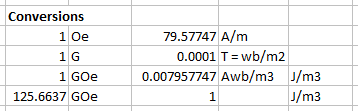So, 125.66 GOe is equal to 1 Joule per cubic meter.

How do we estimate the area under the curve for the Alnico-5 magnet?  Break it up into rectangles and triangles and sum the areas.
The area of a rectangle is the base x height.  The area of a triangle is 1/2 x base x height.

Here's how I broke it up into those areas.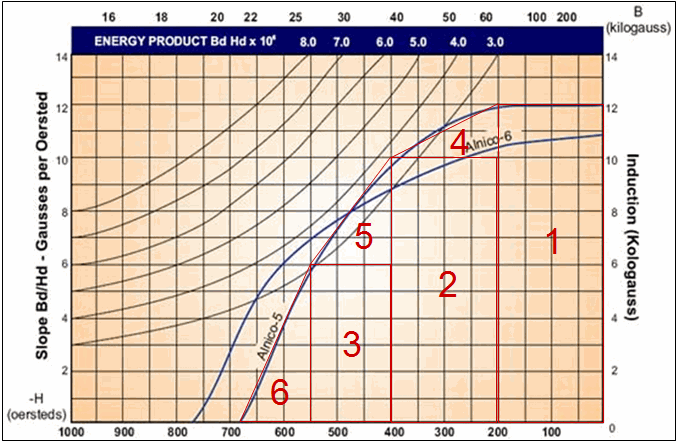Summing the areas and doing the conversion:So we get about 50 kJ/m3

If we take a bar magnet that measures 6" long by 1" wide by 1/4" thick, we would have a volume of: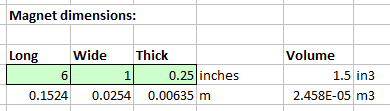The energy stored then is 49,258 J/m3 * 0.00002458 m3 = 1.21 Joules.

Here is a typical B-H curve for a NdFeB magnet (rare-earth magnet, neodymium-iron-boron magnet).
Notice how its coercivity is much, much greater than Alnico.  These are hard to demagnetize.  Alnico is easy to demagnetize.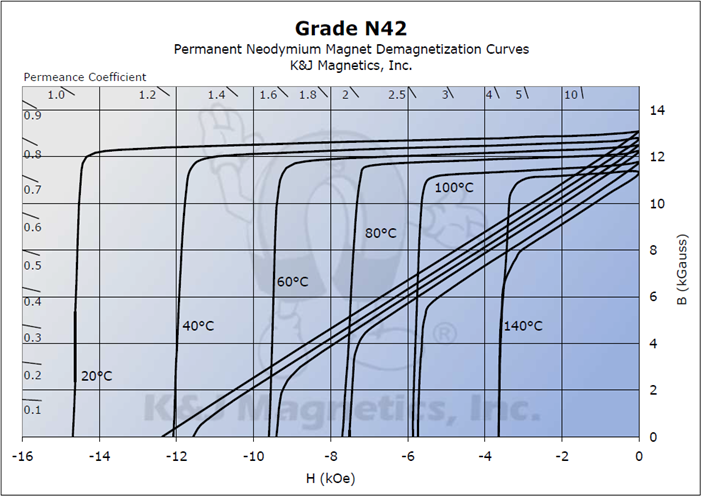This is easier to find the area since we have a big triangle.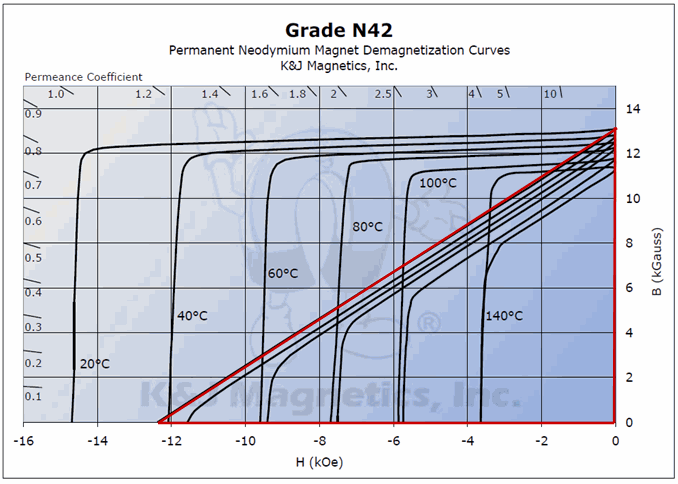The area is: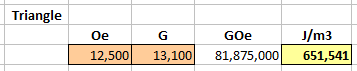So we get about 650 kJ/m3

If we take a bar magnet with the same measurements of 6" long by 1" wide by 1/4" thick, then ...

The energy stored then is 651,541 J/m3 * 0.00002458 m3 = 16.02 Joules.

That is 13.2 times as much energy as the Alnico magnet!

This is enough energy to keep a 100W (100 Joules per second) lightbulb lit for about 160 milliseconds.

However, an AA alkaline battery stores about 15,400 Joules of energy, about 1000 times as much as that bar magnet.

Let's compare this to other items.  To do this, we will need to convert m3 to kg for this material.

1 cubic centimeter of NdFeB = 7.5 g
1 cubic meter = 7,500,000 g = 7,500 kg  (about 16,535 pounds or ~8 tons!)
So, 651,541 J/m3 * 1 m3/7,500 kg = 86.9 J/kg of NdFeB N42 magnet material.

Looking at the Specific Energy of various materials, we get: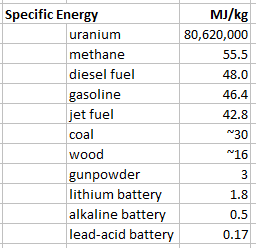A NdFeB magnet can pack a whopping 0.0000869 MJ/kg.  Not much.

In order to magnetize a magnet, it typically takes twice as much energy as it stores, since some energy is wasted as heat.
http://www.dextermag.com/resource-center/magnetization-demagnetization-faq#2